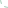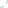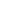Quilting > Ask Margarit Archives > Fall 2006

The e-Forum column is dedicated to you, the quilter. I would like to encourage you to participate in the e-Forum by submitting any events, thoughts, questions and hints to "Ask Margrit" and submitting your information via e-mail. If you submit information regarding quilting events in your area, we will post as many as possible. Please submit at least 2 months prior to the event.

In this edition of Ask Margrit:

The Following two questions are representative of a number of similar questions that I receive on an on going basis. Hopefully the answers will help you make those perfect fitting triangles.

Q:  I am pulling my hair out trying to figure out how to cut triangles to make a square in a square. Is there a magic formula? I have already ruined a lot of nice fabric.

A:  To figure the size of the triangles to fit on the square, you take the finished size of the side of the square and divide by 1.414, round up to nearest 1/8" and the add 7/8 (.875) to that measurement. Cut a square that size and cut once across the diagonal to make 2 triangles. Example:

Finished size of square is 4. Divide 4 by 1.414 to equal 2.828, round up to 2.875 (2 7/8) add 7/8" for a total of 3.75" (2 3/4"). Cut a square 3 3/4" and cut once across the diagonal. You will now have a triangle that will fit on to the side of the square. You need two 3 3/4" squares, cut once across the diagonal for each square in a square.

Q:  I am making a nine patch quilt set on point.  The patches are 8" square.  I would like to know if there is a formula for making the triangles that go onto the ends to make the quilt square.  I'm not sure what size to make them.  I would appreciate any help you can give me.

A:  To figure the set in triangles, multiply the finished size of the square (the square size minus the 1/2" seam allowances). If the finished square is 7 1/2" (7.5), multiply 7.5 x 1.414 = 10.605. Round up to the nearest 1/8" to 10.625 (10 5/8") and add 1.25" (10.625 + 1.25 = 11.875 (11 7/8"). Cut the square 11 7/8" x 11 7/8". Cut the square across both diagonals. The resulting triangles are your set in triangles.

When you cut a square across both diagonals they are called quarter (1/4) square triangles. (4 triangles per square with the straight of grain a long the long side of the triangle)

To figure your 4 corner triangles divide the finished square size by 1.414 (7.5 ÷ 1.414 = 5.304 round up to the nearest 1/8" to 5.375 or 5 3/8"). Add 5.375 + .875 = 6.25 (6 1/4"). Cut a square 6 1/4" x 6 1/4". Cut the square once across a diagonal.

When you cut a square once across the diagonal they are called half (1/2) square triangles (2 triangles per square with the straight of grain on the short sides of the triangle)

Attached is a 1/2 and 1/4 square triangle chart with a fraction and decimal conversion table.

Half and Quarter Square Triangles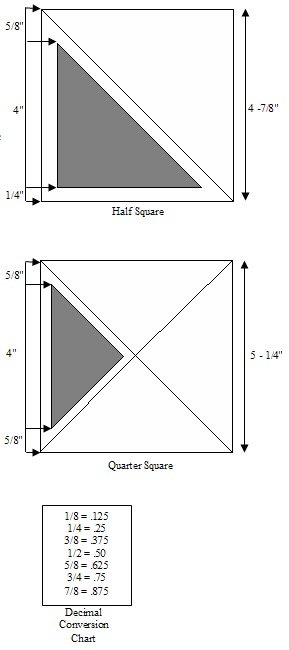To the finished measurement of the short side of the triangle add 7/8".

Finished Side = 4"
4" + 7/8" = 4-7/8"
Block Finished Size + 7/8" = Size of Square

To the finished measurement of the long side of the triangle add 1-1/4".

Finished Side = 4"
4" + 1-1/4" = 5-1/4"
Block Finished Size + 1-1/4" = Size of Square

To convert a half square triangle to a quarter square triangle, multiply the finished measurement of the short side of the triangle by 1.414 and add 1-1/4".

Example: 4" x 1.414 = 5.656" + 1-1/4" = 6.906 or 7" (rounded to the next highest 1/8")

To convert a quarter square triangle to a half square triangle, divide the finished measurement of the long side of the triangle by 1.414 and add 7/8".

Example: 4" ÷ 1.414 = 2.828" + 7/8" = 3.70 or 3-3/4"

Q:  I have a quick quilting question and I'd be so obliged if you could answer. Is it possible to use eyelet to make up quilt blocks, or will the batting show through or come through the holes? I am designing a very delicate-looking spring quilt and would love to employ eyelet in a few of the 9 inch blocks, but am worried about how it will work out. Have you ever seen this done? I'd so appreciate your response!

A:  If you line the eyelet with a cotton batiste (it is a light weight cotton used in French hand sewing) it will have the same feel as the eyelet and the batting will not show through. Simply baste the cut edge of the eyelet to a piece of batiste (the same color as the eyelet) that is cut the same width as the eyelet and then use it as through it is one piece of fabric.

Q:  I am learning to machine appliqué and using a blanket stitch, but my thread will start fraying and break after a little while what do I need to do?

A:  I checked with Rose Hughes, an expert in machine appliqué and embroidery. She said it is usually one of three things.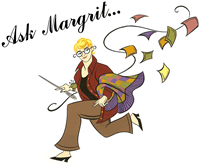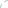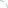Archived columns: February 2009 January 2009 Spring 2008 Winter 2008 Fall 2007 Summer 2007 Spring 2007 Winter 2007 Late Fall 2006 Fall 2006 Spring/Summer 2006 Winter 2006 Late Fall 2005 Fall 2005 Late Summer 2005 Summer 2005 Spring 2005 Fall/Winter 2004